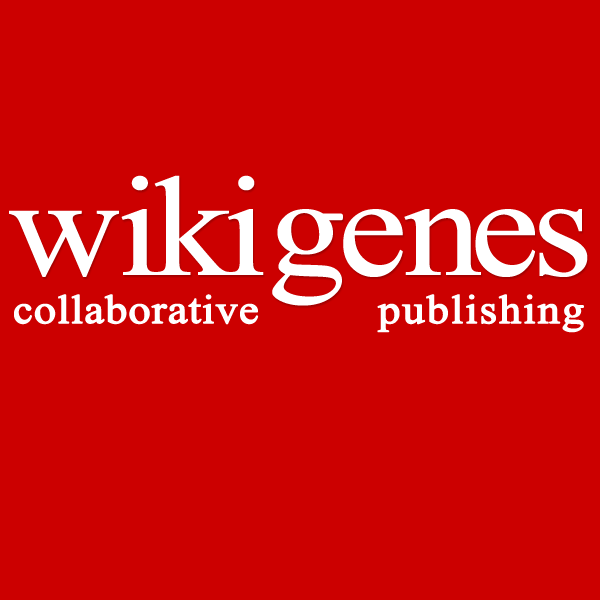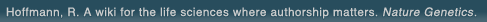# The world's first wiki where authorship really matters (Nature Genetics, 2008). Due credit and reputation for authors. Imagine a global collaborative knowledge base for original thoughts. Search thousands of articles and collaborate with scientists around the globe.

wikigene or wiki gene protein drug chemical gene disease author authorship tracking collaborative publishing evolutionary knowledge reputation system wiki2.0 global collaboration genes proteins drugs chemicals diseases compound
Hoffmann, R. A wiki for the life sciences where authorship matters. Nature Genetics (2008)# The possible meaning of transferrin and its soluble receptors in seminal plasma as markers of the seminiferous epithelium.

Transferrin (Tf) and soluble transferrin receptors (S-Tf-R) were measured by enzyme immunoassay in seminal plasma of 130 semen samples. The mean concentration of S-Tf-R in cases with normozoospermia was 10.4 IU/ml (95% confidence interval: 9.5-11.3) and it was significantly lower in patients with oligozoospermia (6.6, 95% CI: 5.8-7.5, P < 0.001), asthenozoospermia (8.5, 95% CI: 5.5-10.7, P < 0.05), azoospermia of primary testicular origin (7.9, 95% CI: 6.1-9.6, P<0.05) and post-vasectomy samples (5.9, 95% CI: 5.4-6.9, P < 0.001). The concentration of S-Tf-R in post-vasectomy samples was lower than that in patients with azoospermia of primary testicular origin (P < 0.05; positive likelihood ration = 7 at value of 8.3 IU/ ml). S-Tf-R was positively correlated with motile sperm concentration (r = 0.50, P < 0.0001), percentage motility (r = 0.38, P < 0.001), percentage of normal forms (r = 0.43, P < 0.001), sperm linear velocity (r = 0.42, P < 0.001), and ATP concentration (r = 0.67, P < 0.0001). Follicle stimulating hormone (FSH) was found to be negatively correlated with the concentrations of both Tf (r = -0.31, P < 0.05) and of S-Tf-R (r = -0.45, P < 0.01). The mean concentration of Tf in seminal plasma was 50.4 micrograms/ml (35.9-67.2) in samples with normozoospermia (n = 22), and the concentration was significantly lower in patients with oligozoospermia (P < 0.05), azoospermia of testicular origin (P < 0.001), and post-vasectomy samples (P < 0.001). Seminal Tf was correlated with motile sperm concentration (r = 0.36, P < 0.001), percentage of motile spermatozoa (r = 0.25, P < 0.05), linear velocity (r = 0.24, P < 0.05) and ATP concentration (r = p.44, P < 0.001). The concentration of Tf was positively correlated with that of S-Tf-R both in cases with spermatozoa present (r = 0.66, P < 0.001), and in cases with azoospermia of testicular origin (r = 0.51, P < 0.05) but not in vasectomy cases. It is concluded that S-Tf-R in seminal plasma is a marker of spermatogenesis and may give information on the presence or absence of spermatogenetic cells in cases with azoospermia. Further investigations are needed to assess its usefulness for clinical practice.